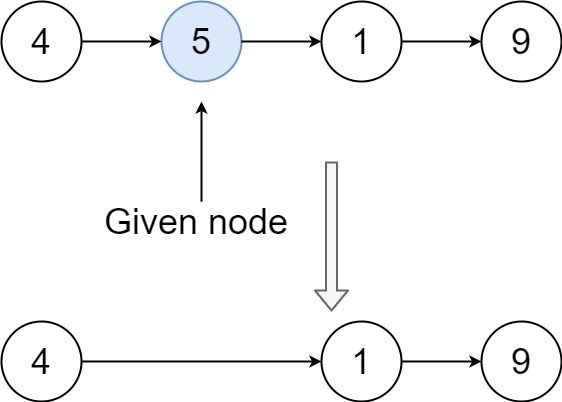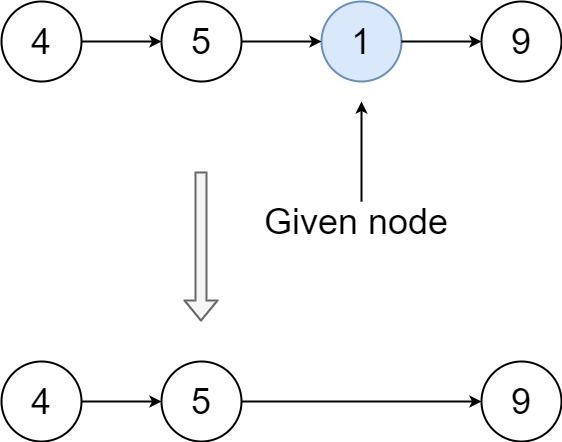# Question

Formatted question description: https://leetcode.ca/all/237.html

There is a singly-linked list head and we want to delete a node node in it.

You are given the node to be deleted node. You will not be given access to the first node of head.

All the values of the linked list are unique, and it is guaranteed that the given node node is not the last node in the linked list.

Delete the given node. Note that by deleting the node, we do not mean removing it from memory. We mean:

• The value of the given node should not exist in the linked list.
• The number of nodes in the linked list should decrease by one.
• All the values before node should be in the same order.
• All the values after node should be in the same order.

Custom testing:

• For the input, you should provide the entire linked list head and the node to be given node. node should not be the last node of the list and should be an actual node in the list.
• We will build the linked list and pass the node to your function.
• The output will be the entire list after calling your function.

Example 1:Input: head = [4,5,1,9], node = 5
Output: [4,1,9]
Explanation: You are given the second node with value 5, the linked list should become 4 -> 1 -> 9 after calling your function.


Example 2:Input: head = [4,5,1,9], node = 1
Output: [4,5,9]
Explanation: You are given the third node with value 1, the linked list should become 4 -> 5 -> 9 after calling your function.


Constraints:

• The number of the nodes in the given list is in the range [2, 1000].
• -1000 <= Node.val <= 1000
• The value of each node in the list is unique.
• The node to be deleted is in the list and is not a tail node.

# Algorithm

First overwrite the value of the current node with the value of the next node, and then we delete the next node.

# Code

• 

/**
* public class ListNode {
*     int val;
*     ListNode next;
*     ListNode(int x) { val = x; }
* }
*/
class Solution {
public void deleteNode(ListNode node) {
node.val = node.next.val;
node.next = node.next.next;
}

}
}

############

/**
* public class ListNode {
*     int val;
*     ListNode next;
*     ListNode(int x) { val = x; }
* }
*/
class Solution {
public void deleteNode(ListNode node) {
node.val = node.next.val;
node.next = node.next.next;
}
}

• // OJ: https://leetcode.com/problems/delete-node-in-a-linked-list/
// Time: O(1)
// Space: O(1)
class Solution {
public:
void deleteNode(ListNode* node) {
node->val = node->next->val;
auto del = node->next;
node->next = del->next;
delete(del);
}
};

• # Definition for singly-linked list.
# class ListNode:
#     def __init__(self, x):
#         self.val = x
#         self.next = None

class Solution:
def deleteNode(self, node):
"""
:type node: ListNode
:rtype: void Do not return anything, modify node in-place instead.
"""
node.val = node.next.val
node.next = node.next.next

############

# class ListNode(object):
#     def __init__(self, x):
#         self.val = x
#         self.next = None

class Solution(object):
def deleteNode(self, node):
"""
:type node: ListNode
:rtype: void Do not return anything, modify node in-place instead.
"""
node.val = node.next.val
node.next = node.next.next


• /**
* type ListNode struct {
*     Val int
*     Next *ListNode
* }
*/
func deleteNode(node *ListNode) {
node.Val = node.Next.Val
node.Next = node.Next.Next
}

• /**
* function ListNode(val) {
*     this.val = val;
*     this.next = null;
* }
*/
/**
* @param {ListNode} node
* @return {void} Do not return anything, modify node in-place instead.
*/
var deleteNode = function (node) {
node.val = node.next.val;
node.next = node.next.next;
};


• /**
* class ListNode {
*     val: number
*     next: ListNode | null
*     constructor(val?: number, next?: ListNode | null) {
*         this.val = (val===undefined ? 0 : val)
*         this.next = (next===undefined ? null : next)
*     }
* }
*/

/**
Do not return anything, modify it in-place instead.
*/
function deleteNode(node: ListNode | null): void {
node.val = node.next.val;
node.next = node.next.next;
}